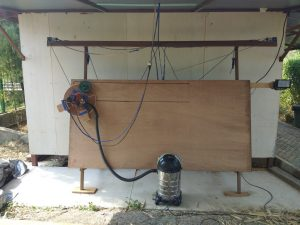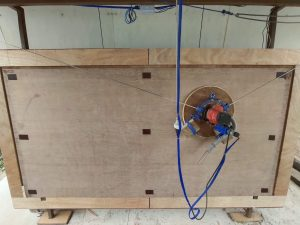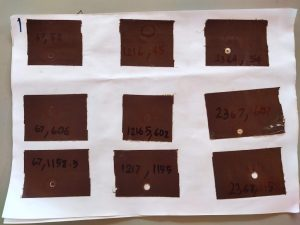We found the problem on the calibration of our Maslow CNC when running some test cuts. While cutting horizontal lines, it curves down about 1-2 cm in the center, even when we set the correct measured distances for the CNC in the “groundcontrol.ini”file.Horizontal straight line curved down.
This really isn’t a surprise. We’ve done a lot of work in the past in colorspace calibration of optical devices, and as a general rule, when you are calibrating, you need to run tests, measure the results, and adjust the input parameters to achieve the desired output results. The Maslow CNC calibration seems to be oriented only towards measuring the input parameters carefully and hoping that the output is what you want. The “closed loop calibration” approach of feeding errors in the output back into adjust input parameters has always worked well for us in other fields, so we decided to implement it here ourselves.

So we created a “refine_calibration_parameters.py” script to adjust the input parameters based on measured output results. To be specific, the script works as follows:

1. First we calibrate as well as possible by measuring everything carefully in order to get the machine within a centimeter or two of calibrated over the entire area.
2. We created a simple GCode file that tells the CNC to go to a number of known points and make a mark on the surface. Any set of scattered points would do, so we did a 3×3 grid of left,middle,right columns and upper,middle,lower rows as follows:|
0    1    2
3    4    5
6    7    8
To avoid wasting wood, we simply put tape on the surface of the wood close to where the expected marks are and told the CNC to just touch the surface. The marks were easily visible in the tape with no noticeable marks on the wood.We put the tape on 9 expected positions on wood.
3. After running the GCode file, we measured the distance from left and top of the edge of the plywood to each of the 9 marks that the CNC made. This gives us a pairing between where the CNC thought it was going to make the mark and where it actually made it. This information (where the GCode told it to go vs where it actually went for each of the 9 marks) was put into a file called “Calibration Measurements.ini” for our calibration script.
4. The script we wrote then reads the “groundcontrol.ini” CNC configuration file used to cut the GCode file as well as the information on target vs actual from “Calibration Measurements.ini”.
5. An interesting little twist is that it made less sense to tweak the actuals to be closer to the target (because this would require running a new cut to check). Rather we compute the chain lengths that the CNC would have used given the “groundcontrol.ini” file configuration at the time of cut to achieve the given targets, and then say “how should we tweak the configuration so that those cable lengths would have corresponded to the actually measured values?” In other words, we tweaked the targets to the actuals given the chain lengths that were actually used for the calibration marks. This can be done thousands of times per second without running any additional cuts on the machine.
6. This is done using a standard multi-variable optimization approach. We create a cost function (sum square Euclidean distance between target points and actual points) and then use Gauss-Newton to adjust the configuration parameters to minimize the cost function (i.e. make the target and actual points line up). The main parameters that we optimized are:
MotorSpacingX = The horizontal distance between the center of the motor shafts in mm
MotorOffsetY = The vertical distance between the motor mounts and the zero position on the cutting bed
RotationDiskRadius = The distance between where the chains attach to the sled and the center of the router bit in mm
ChainSagCorrection = A single parameter to model the amount of chain sag that occurs when the chain is mostly horizontal. One would expect this to be affected by the sled weight and chain weight.
7. We added a few additional calibration parameters like the horizontal offset of the motors relative to the cutting bed, as well as an angular offset between the line connecting the 2 motors and the cutting bed. Personally, I would have included these as calibration parameters in the MaslowCNC firmware, but they didn’t. These values are very small by design of the machine, but still adding them to the mathematical model allowed the optimizer to more accurately compute the above calibration parameters.
8. Finally, after minimizing for a few seconds, the script produces a new “groundcontrol.ini” file that replaces the optimized parameters in order to better achieve the lineup of actual and target positions.

You can replace the input “groundcontrol.ini” with the output and run the calibration again to see how much it improved.

Here is before calibrating:Before calibration result, middle column shifted down about 1 cm.

And here is after the first 2 iterations:

Updated :
“RefineCalibrationParameters” script were merged to Master in GroundControl on the MaslowCNC github repo.

The “Calibration Measurements.ini” file example was pasted down here. feel free to copy and change the actual position referenced from top-left in mm unit.

``[global]numpositions = 9[actual_positions]x0 = 67y0 = 52x1 = 1216y1 = 45x2 = 2364y2 = 54###x3 = 67y3 = 606x4 = 1216y4 = 602x5 = 2367y5 = 607###x6 = 67y6 = 1158.5x7 = 1217y7 = 1155x8 = 2368y8 = 1158[target_positions]tx0 = 69.2ty0 = 59.6tx1 = 1219.2ty1 = 59.6tx2 = 2369.2ty2 = 59.6###tx3 = 69.2ty3 = 609.6tx4 = 1219.2ty4 = 609.6tx5 = 2369.2ty5 = 609.6###tx6 = 69.2ty6 = 1159.6tx7 = 1219.2ty7 = 1159.6tx8 = 2369.2ty8 = 1159.6``